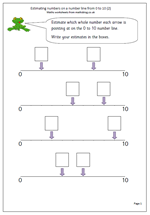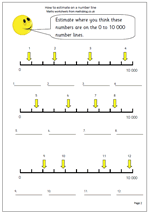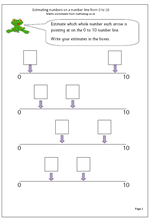## Year 2: estimating on a number line 0-10By year 2 children will be beginning to understand the vocabulary of estimating, which includes other terms such as roughly, guess how many, about the same as, nearly, close to etc.

This worksheet looks  at estimating a whole number on a number line from 0 to 10. Probably the best technique to use is to try and estimate the middle of the line, which will be 5 and then work either from 0 to 5 or 5 to 10. This is not as easy as it might appear, but after a few goes it does become much less tricky.

One use for this type of activity will come later when children need to interpret graphs where the numbers on the axes go up in fours, fives or tens and intermediate numbers need to be found.

Estimating on a number line (2)

## How to estimate on a 0-10 000 number lineThis is a much trickier exercise than it first appears. Firstly, children need to be confident with reading large numbers and counting in thousands. Secondly they need to be able to visualise marks on a scale.

Only the zero and 10 000 are marked on the number line so it would be a good idea to jot down each of the thousands before trying to estimate where the arrows are pointing.

Then it is a good idea to work out if the arrow is pointing above or below the half way mark and then make an intelligent estimate according to this.

How to estimate on a number line (1)

## Maths worksheet: estimating on a number lineBy year 2 children should be beginning to understand the vocabulary of estimating, which includes other terms such as roughly, guess how many, about the same as, nearly, close to etc.

This worksheet looks  at estimating a whole number on a number line from 0 to 10. Probably the best technique to use is to try and estimate the middle of the line, which will be 5 and then work either from 0 to 5 or 5 to 10.

Estimating on a number line (pg 1)